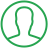# Python Count Frequency Of Characters In String

Last Updated : Jul 1, 2023In this article we will show you the solution of python count frequency of characters in string, create a Python programme to count the instances of each character in the string, which represents the character's frequency.

After being given the string, the objective is to determine the frequency of every letter in it.

The character is the key, as well as the frequency of all that character is the value, in a dictionary.

A character's frequency within a string can be counted using the count() method.

The count() method accepts a character as an argument when it is called on a string. Let's talk about the idea of Python character frequency counting.

## Step By Step Guide On Python Count Frequency Of Characters In String :-

### Method - using in operator

```str1 = input ("Enter the string: ")
d = dict()
for c in str1:
if c in d:
d[c] = d[c] + 1
else:
d[c] = 1
print(d)```
1. As you can see, we used the in operator to build the Python code of counting the frequency of characters.
2. Start by making a string variable, then take the user's string and save it in the variable. Here, the variable str1 is a string.
3. Then, make an empty dictionary and enter the character as the key and the character's frequency as the value.
4. To extract each character individually, loop through the string (each iteration).
5. The in operator can be used to determine whether a character already is present in the dictionary.
6. Add the character, with the character as the key and the value set to 1, to the dictionary if it is missing.
7. The character's value should be increased by 1 if it appears in the dictionary already.
8. Print the dictionary once more using the characters as the keys and the values as the character frequency.

### Method - Use of “get()” function

```str1 = input ("Enter the string: ")
d = dict()
for c in str1:
d[c] = d.get(c, 0) + 1
print(d)
```
1. Users are able to observe that we use the get function to construct Python code in this example to count the frequency for characters in a string.
2. Start by making a string variable, then take the user's string and save it in the variable. Here, the variable str1 is a string.
3. Then, make an empty dictionary and enter the character as the key and the character's frequency as the value.
4. To extract each character individually, loop through the string (each iteration).
5. Just use get() method to determine whether a character is present in the dictionary. If it is, it returns the character's value; otherwise, it adds one.
6. The second parameter, which is returned by the get() function if the character is missing, is set to 0 and the character is entered into the dictionary once it has been multiplied by 1.
7. Print the dictionary once more using the characters as the keys and the values as the character frequency.

## Conclusion :-

As a result, we were able to understand the idea of the Python count frequency for characters in string. We also discovered that the get() function requires two parameters.

It determines whether the first parameter, which serves as the dictionary's key, is present. If it is, it returns the key's value; otherwise, it returns zero.

I hope this article on python count frequency of characters in string helps you and the steps and method mentioned above are easy to follow and implement.

##About Dikshita

Passionate Electronics and Communication Engineering student with expertise in web development (HTML, CSS, JS,PHP, Bootstrap, React.js) and content writing. Eager problem solver and tech enthusiast, adept at creating engaging web experiences.